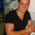# Programmed responses

Question types ::: qtype_programmedresp
Maintained byGerard Cuello Adell
Question type which gets the question answer through a set of editable and non-native PHP functions. Useful for maths or statistics exercices which requires complex operations to get the answer.
Latest release:
3 sites
5 fans
Current versions available: 1

FEATURES
* Users can add variables to the question text, the variables takes random values depending on
the user selection: max value, min value, increment and the number of values (scalar/vectorial - float/array)

* The function arguments can be one of the question text defined variables, a concatenation of defined variables or fixed values.

* User interface to allow the addition of functions. These functions can return one or more values, useful for example to return confidence intervals.

USAGE
#1st step (optional): Variables can be added to the question text following the next format: {\$varname}
The variables only accepts alphanumeric characters.

#2nd step (optional, depends on the first step): Each variable must define the maximum and minimum values it can take, the increment and the number of values, to allow vectorial variables.

#3rd step (optional): Add concat variables, useful if there should be a vectorial variable which values follows different criteria

#5th step: Assign each of the function arguments to a variable or a concat variable, the function can also use a fixed value as argument

There is an interface to allow the addition of functions; preceding the php implementation of each function there should be a comment block following phpdoc format, to specify the arguments description and the returned values.

### Contributors